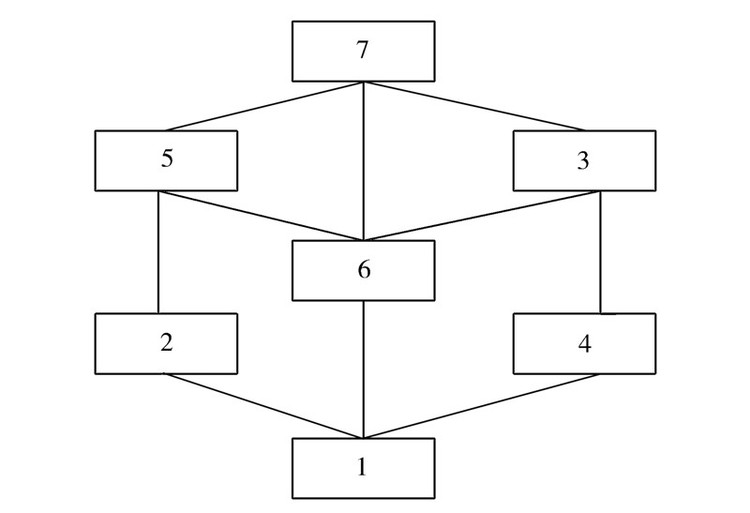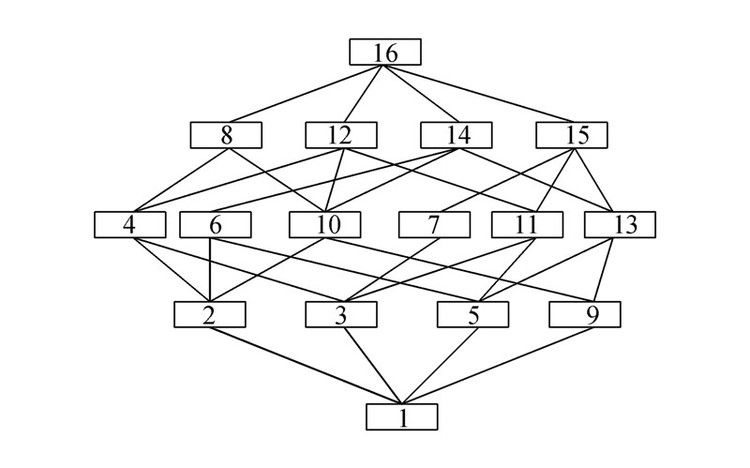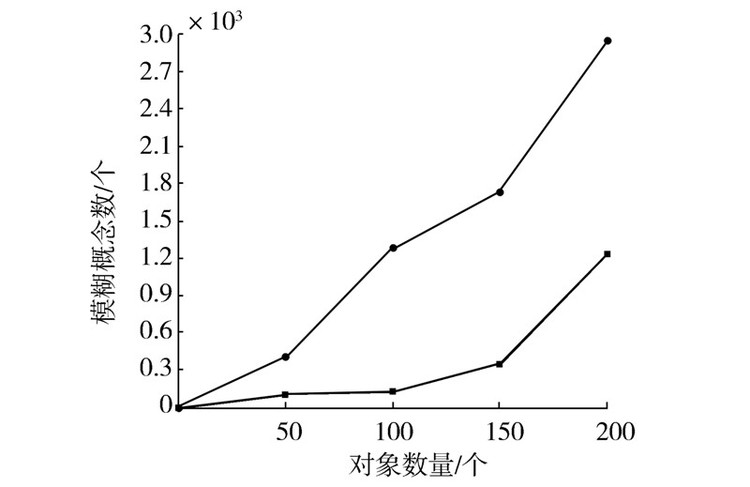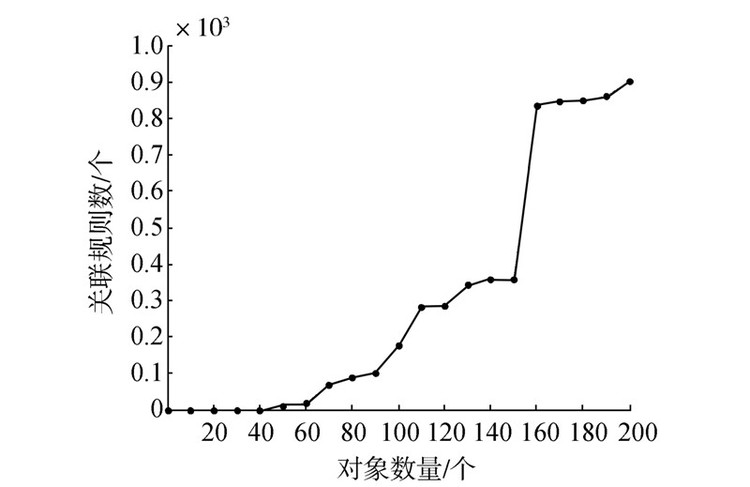Download PDF文章快速检索 高级检索

Research on rule extraction method based on compatibility fuzzy concept
HU Xiaokang, WANG JunhongSchool of Computer and Information Technology, Shanxi University, Taiyuan 030006, China
Abstract: The concept lattice is an effective data analysis and rule extraction tool with a strict mathematical model. In most instances, studies are carried out in a complete formal context, i.e., a two-value context. However, in real life, an incomplete fuzzy formal context is frequently experienced. Incomplete fuzzy contexts contain a lot of uncertain information. There are both distinctions and relationships that can be identified between the forms of knowledge representation in the incomplete fuzzy formal and complete formal contexts. To study their internal relationship, in this paper, we define approximate fuzzy and compatible fuzzy concepts, establish an approximate fuzzy concept lattice, and identify a partial ordering relationship between compatible fuzzy concepts in an incomplete fuzzy formal context. We extend the design of an association rules mining algorithm to address the background of the incomplete fuzzy formal context, and conduct an experiment to demonstrate the feasibility and effectiveness of the proposed method.
Key words: formal context     concept lattice     approximate fuzzy concept     compatible fuzzy concept     knowledge representation     association rules     partial ordering relation     compatible relation
﻿

1 基本概念 1.1 形式概念分析

 $F(A)=\{m\in M|gIm,\forall g\in A\},A\subseteq G$ (1)
 $G(B)=\{g\in G|gIm,\forall m\in B\},B\subseteq M$ (2)

 G a b c d X1 1 0 0 1 X2 0 1 1 0 X3 1 0 1 0 X4 0 0 1 0图 1 表 1对应概念格的Hasse图 Fig. 1 Hasse diagram of table 1

1){∅,(a,b,c,d)}；

2){(x1),(a,d)}；

3){(x3),(a,c)}；

4){(x2),(b,c)}；

5){(x1,x3),(a)}；

6){(x2,x3,x4),(c)}；

7){(x1,x2,x3,x4),∅}。

 $\text{FA}(A)=\{m\in M'|\forall g\in A:{{t}_{1}}\le u(g,m)\le {{t}_{2}}\}$ (3)

 $\text{FO}(B)=\{g\in G'|\forall m\in B:{{t}_{1}}\le u(g,m)\le {{t}_{2}}\}$ (4)

 a b c d e f o1 0.8 0.1 0.61 0.6 0.8 * o2 0.9 0.85 * 0.2 0.7 0.9 o3 0.21 * 0.87 * 0.6 0.6 o4 0.6 * 0.30 * 0.5 0

2 相似模糊概念与相容模糊概念

1)删除法。删除法即删除形式背景中缺失数据的一列或者一行，也就是删除一个对象或者删除一个属性。这类方法操作起来比较简单，但是在删除过程中会导致原先存在的数据缺失，可能会造成获取的知识不准确。

2)填补法。填补法就是对不完备形式背景中缺失的数据填充为1或者0，使之补全为一个完备的形式背景。这类方法比较简单，但是容易造成获取的知识错误，因为好多缺失信息都是人为地填充0或者1。

3)扩展属性法。扩展属性法即把原有不完备形式背景下的属性集合中的属性分为完备和不完备属性两部分，然后将不完备属性在不同对象的不同取值进行扩展，从而把不完备形式背景补充完整。此方法的好处是既不会增加知识也不会缺失知识，但是增加了知识获取的时间和空间复杂度。

 \begin{align} & \underline{FA(A)}=\{m\in M'|\forall g\in A:{{t}_{1}}\le \\ & u(g,m)\le {{t}_{2}}或u(g,m)=*\} \\ \end{align} (5)

 \begin{align} & \underline{FO(B)}=\{g\in G'|\forall m\in B:{{t}_{1}}\le \\ & u(g,m)\le {{t}_{2}}或u(g,m)=*\} \\ \end{align} (6)

 ${{u}_{g}}=\min (u(g,m))m\in B$ (7)

 $\chi (A,B)=\{a\in B|\left| A,B{{)}_{a}} \right|\ge \alpha \times \left| A \right|\}$ (8)
 $\varphi (A,B)=\{x\in A|\left| A,B{{)}^{x}} \right|\ge \alpha \times \left| B \right|\}$ (9)
 $\gamma (A,B)=\frac{1}{|A|+|B|}(|\chi (A,B)\left| + \right|\varphi (A,B)|)$ (10)

 $\bar{u}=\frac{\sum\limits_{g\in A}{{{u}_{g}}}}{|A|}$ (11)

 $\frac{|u{{(g,m)}_{(\forall g\in A)}}=*|}{|A|\times |B|}$ (12)

1)先对不完备模糊形式背景进行处理，如果u(g,m)小于置信度阈值T，则u(g,m)为0，然后将Kc中的空缺数据*全部填充为0.5，即用补全法把不完备形式背景转化为完备形式背景。

2)获得第一个概念(FO(M),M)设置概念的隶属度值并加入w(Kc)中。

3)遍历对象g，其中gG，如果遍历完成转到6)，反之转到4)。

4)遍历近似模糊概念(A,B)，其中(A,B)∈wKc，如果遍历完成转到3)，否则转到5)。

5)求出BFA(g)交集I，如果获得的交集I不是已获得w(Kc)的内涵，则计算出(FO(I),I)隶属度值并加入w(Kc)中然后回到4)。

6)输出w(Kc)，算法结束。

1)任取wKc里的相似模糊概念并计算出χ(A,B)、φ(A,B)与γ(A,B)。如果γ(A,B)>β，计算出(A,B)的隶属度值u并加入到相容模糊概念wβα(Kc)中。

2)如果相似模糊概念都被进行计算过，则输出相容模糊概念转到3)，反之再进行1)。

3)输出wβα(Kc)，算法结束。

3 基于相容模糊概念的规则提取

1)遍历相似模糊概念(A,B)，其中(A,B)∈w(Kc)，并且设置(A,B)的count为0。如果遍历完成则转4)，否则转2)。

2)遍历属性m，其中mM，并求得AFO(m)交集I。如果遍历结束转到1)，否则转到3)。

3)在wKc找出相似模糊概念A1,B1使得A1=I，并把概念A1,B1的 count值加1。假如|B1|-|B|等于A1,B1的count值，则增加边在A1,B1与(A,B)，反之转2)。

4)输出相似模糊概念格，算法结束。

1)对相似模糊概念格进行处理，除去相容模糊概念之外的概念，更新父节点和子节点。

2)如果概念C1=A1,B1C2=A2,B2满足C2C1的父节点，且满足C1C2的隶属度大于给定的阈值η，即$\frac{\min (\bar{u}({{A}_{1}},{{B}_{1}}),\bar{u}({{A}_{2}},{{B}_{2}}))}{\max (\bar{u}({{A}_{1}},{{B}_{1}}),\bar{u}({{A}_{2}},{{B}_{2}}))}>\eta$，则可以将得到的规则B2=B1-B2加入∑中，其可信度是|A1|/|A2|。

3)如果对于节点C=A,B有多个双亲节点，则任取两个双亲节点C1=A1,B1C2=A2,B2，如果满足条件$\frac{\min (\bar{u}({{A}_{1}},{{B}_{1}}),\bar{u}({{A}_{2}},{{B}_{2}}))}{\max (\bar{u}({{A}_{1}},{{B}_{1}}),\bar{u}({{A}_{2}},{{B}_{2}}))}>\eta$，则可以提取到的规则B1B2B2B1，并将其加入∑中，支持度分别为|A|/|A1|与|A|/|A2|。

4)输出∑。

4 示例展示

 a b c d e f o1 0.8 0 0.61 0.6 0.8 * o2 0.9 0.85 * 0 0.7 0.9 o3 0 * 0.87 * 0.6 0.6 o4 0.6 * 0 * 0.5 0

1){⇒,(a,b,c,d,e,f)}；

2){(0.6/o1),(a,c,d,e,f)}；

3){(0.5/o2),(a,b,c,e,f)}；

4){(0.61/o1,0.5/o2),(a,c,e,f)}；

5){(0.5/o3),(b,c,d,e,f)}；

6){(0.6/o1,0.5/o3),(c,d,e,f)}；

7){(0.5/o2,0.5/o3),(b,c,e,f)}；

8){(0.61/o1,0.5/o2,0.6/o3),(c,e,f)}.；

9){(0.5/o4),(a,b,d,e)}；

10){(0.6/o1,0.5/o4),(a,d,e)}；

11){(0.7/o2,0.5/o4),(a,b,e)}；

12){(0.8/o1,0.7/o2,0.5/o4),(a,e)}；

13){(0.5/o3,0.5/o4),(b,d,e)}；

14){(0.6/o1,0.5/o3,0.5/o4),(d,e)}；

15){(0.7/o2,0.5/o3,0.5/o4),(b,e)}；

16){(o1,o2,o3,o4),(0/a,0/b,0/c,0/d,0.5/e,0/f)}。图 2 相似模糊概念的相似模糊概念格 Fig. 2 Approximat fuzzy concept lattice

1){0.6/(o1),(a,c,d,e,f)}；

2){0.5/(o2),(a,b,c,e,f)}；

3){0.57/(o1,o2,o3),(c,e,f)}；

4){0.55/(o1,o4),(a,d,e)}；

5){0.6/(o2,o4),(a,d,e)}；

6){0.667/(o1,o2,o4),(a,e)}；

7){0.083/(o1,o2,o3,o4),(c)}。

1){a,d,e}⇒{c,f}置信度为0.5。

2){a,e}⇒{b}置信度为0.67。

5 实验结果与分析图 3 对象与概念个数关系 Fig. 3 Relationship between object and concept图 4 对象与关联规则个数关系 Fig. 4 Relationship between object and association rule
6 结束语

  WILLE R. Restructuring lattice theory: an approach based on hierarchies of concepts[M]//RIVAL I. Ordered sets. Netherlands: Springer, 1982: 445-470.  POELMANS J, IGNATOV D I, KUZNETSOV S O, et al. Formal concept analysis in knowledge processing: a survey on applications[J]. Expert systems with applications, 2013, 40(16): 6538-6560.  MINEAU G W, GODIN R. Automatic structuring of knowledge bases by conceptual clustering[J]. IEEE transactions on knowledge and data engineering, 1995, 7(5): 824-829.  COLE R, EKLUND P W. Scalability in formal concept analysis[J]. Computational intelligence, 1999, 15(1): 11-27.  CARPINETO C, ROMANO G. A lattice conceptual clustering system and its application to browsing retrieval[J]. Machine learning, 1996, 24(2): 95-122.  MA Jianmin, ZHANG Wenxiu. Axiomatic characterizations of dual concept lattices[J]. International journal of approximate reasoning, 2013, 54(5): 690-697.  胡明涵, 张莉, 任飞亮. 模糊形式概念分析与模糊概念格[J]. 东北大学学报:自然科学版, 2007, 28(9): 1274-1277. HU Minghan, ZHANG Li, REN Feiliang. Fuzzy formal concept analysis and fuzzy concept lattices[J]. Journal of northeastern university : natural science, 2007, 28(9): 1274-1277.  GRZYMALA-BUSSE J W. Rough set approach to incomplete data[C]//Proceedings of the 7th international conference on artificial intelligence and soft computing-ICAISC 2004. Berlin Heidelberg, Germany, 2004: 50-55.  LIU Jun, YAO Xiaoqiu. Formal concept analysis of incomplete information system[C]//Proceedings of the 7th international conference on fuzzy systems and knowledge discovery. Yantai, China, 2010, 5: 2016-2020.  DJOUADI Y, DUBOIS D, PRADE H. Possibility theory and formal concept analysis: Context decomposition and uncertainty handling[C]//Proceedings of the 13th international conference on information processing and management of uncertainty. Berlin Heidelberg, Germany, 2010: 260-269.  KRUPKA M. Fuzzy concept lattices with incomplete knowledge[C]//Proceedings of the 14th international conference on information processing and management of uncertainty in knowledge-based systems. Berlin Heidelberg, Germany, 2012: 171-180.  DJOUADI Y, PRADE H. Interval-valued fuzzy formal concept analysis[C]//Proceedings of the 18th international symposium. Berlin Heidelberg, Germany, 2009: 592-601.  LI Jinhai, MEI Changlin, LV Yuejin. Incomplete decision contexts: approximate concept construction, rule acquisition and knowledge reduction[J]. International journal of approximate reasoning, 2013, 54(1): 149-165.  LAI Hongliang, ZHANG Dexue. Concept lattices of fuzzy contexts: formal concept analysis vs. rough set theory[J]. International journal of approximate reasoning, 2009, 50(5): 695-707.  何淑贤, 王育红, 翟岩慧, 等. 不完备形式背景及其完备化方法[J]. 山西大学学报:自然科学版, 2006, 29(4): 364-367. HE Shuxian, WANG Yuhong, ZHAI Yanhui, et al. Incomplete formal context and the completion approach[J]. Journal of Shanxi university : natural science edition, 2006, 29(4): 364-367.  谢志鹏, 刘宗田. 概念格的快速渐进式构造算法[J]. 计算机学报, 2002, 25(5): 490-496. XIE Zhipeng, LIU Zongtian. A fast incremental algorithm for building concept lattice[J]. Chinese journal of computers, 2002, 25(5): 490-496.  梁吉业, 王俊红. 基于概念格的规则产生集挖掘算法[J]. 计算机研究与发展, 2004, 41(8): 1339-1344. LIANG Jiye, WANG Junhong. An algorithm for extracting rule-generating sets based on concept lattice[J]. Journal of computer research and development, 2004, 41(8): 1339-1344.  LEKHA A, SRIKRISHNA C V, VINOD V. Fuzzy association rule mining[J]. Journal of computer science, 2015, 11(1): 71-74.  LAKHAL L, STUMME G. Efficient mining of association rules based on formal concept analysis[M]//GANTER B, STUMME G, WILLE R. Formal concept analysis. Berlin Heidelberg: Springer-Verlag, 2005: 180-195.  KUMAR CH A, DIAS S M, VIEIRA N J. Knowledge reduction in formal contexts using non-negative matrix factorization[J]. Mathematics and computers in simulation, 2015, 109: 46-63.  王志海, 胡可云, 胡学纲, 等. 概念格上规则提取的一般算法与渐进式算法[J]. 计算机学报, 1991, 22(1): 66-70. WANG Zhihai, HU Keyun, HU Xuegang, et al. General and incremental algorithms of rule extraction based on concept lattice[J]. Chinese journal of computers, 1991, 22(1): 66-70.
DOI: 10.11992/tis.201603043

0

#### 文章信息

HU Xiaokang, WANG Junhong

Research on rule extraction method based on compatibility fuzzy concept

CAAI Transactions on Intelligent Systems, 2016, 11(3): 352-358.
DOI: 10.11992/tis.201603043